# plot

Visualize prior and posterior densities of Bayesian linear regression model parameters

## Syntax

``plot(PosteriorMdl)``
``plot(PriorMdl)``
``plot(PosteriorMdl,PriorMdl)``
``plot(___,Name,Value)``
``pointsUsed = plot(___)``
``````[pointsUsed,posteriorDensity,priorDensity] = plot(___)``````
``````[pointsUsed,posteriorDensity,priorDensity,FigureHandle] = plot(___)``````

## Description

example

````plot(PosteriorMdl)` or `plot(PriorMdl)` plots the posterior or prior distributions of the parameters in the Bayesian linear regression model `PosteriorMdl` or `PriorMdl`, respectively. `plot` adds subplots for each parameter to one figure and overwrites the same figure when you call `plot` multiple times.```

example

````plot(PosteriorMdl,PriorMdl)` plots the posterior and prior distributions in the same subplot. `plot` uses solid blue lines for posterior densities and dashed red lines for prior densities.```

example

````plot(___,Name,Value)` uses any of the input argument combinations in the previous syntaxes and additional options specified by one or more name-value pair arguments. For example, you can evaluate the posterior or prior density by supplying values of β and σ2, or choose which parameter distributions to include in the figure.```

example

````pointsUsed = plot(___)` also returns the values of the parameters that `plot` uses to evaluate the densities in the subplots.```

example

``````[pointsUsed,posteriorDensity,priorDensity] = plot(___)``` also returns the values of the evaluated densities.If you specify one model, then `plot` returns the density values in `PosteriorDensity`. Otherwise, `plot` returns the posterior density values in `PosteriorDensity` and the prior density values in `PriorDensity`.```

example

``````[pointsUsed,posteriorDensity,priorDensity,FigureHandle] = plot(___)``` returns the figure handle of the figure containing the distributions.```

## Examples

collapse all

Consider the multiple linear regression model that predicts the US real gross national product (`GNPR`) using a linear combination of industrial production index (`IPI`), total employment (`E`), and real wages (`WR`).

`${\text{GNPR}}_{t}={\beta }_{0}+{\beta }_{1}{\text{IPI}}_{t}+{\beta }_{2}{\text{E}}_{t}+{\beta }_{3}{\text{WR}}_{t}+{\epsilon }_{t}.$`

For all $t$, ${\epsilon }_{t}$ is a series of independent Gaussian disturbances with a mean of 0 and variance ${\sigma }^{2}$.

Assume these prior distributions:

• $\beta |{\sigma }^{2}\sim {N}_{4}\left(M,{\sigma }^{2}V\right)$. $M$ is a 4-by-1 vector of means, and $V$ is a scaled 4-by-4 positive definite covariance matrix.

• ${\sigma }^{2}\sim IG\left(A,B\right)$. $A$ and $B$ are the shape and scale, respectively, of an inverse gamma distribution.

These assumptions and the data likelihood imply a normal-inverse-gamma conjugate model.

Create a normal-inverse-gamma conjugate prior model for the linear regression parameters. Specify the number of predictors `p` and the variable names.

```p = 3; VarNames = ["IPI" "E" "WR"]; PriorMdl = bayeslm(p,'ModelType','conjugate','VarNames',VarNames);```

`PriorMdl` is a `conjugateblm` Bayesian linear regression model object representing the prior distribution of the regression coefficients and disturbance variance.

Plot the prior distributions.

`plot(PriorMdl);`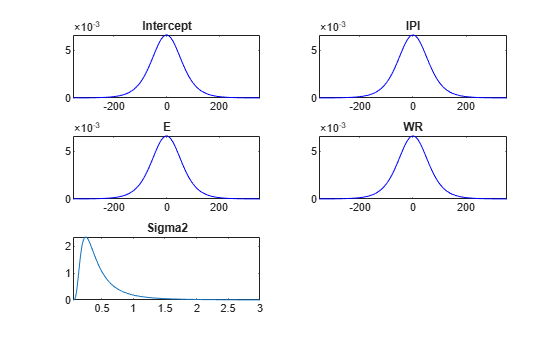`plot` plots the marginal prior distributions of the intercept, regression coefficients, and disturbance variance.

Suppose that the mean of the regression coefficients is $\left[-20\phantom{\rule{0.2777777777777778em}{0ex}}4\phantom{\rule{0.2777777777777778em}{0ex}}0.001\phantom{\rule{0.2777777777777778em}{0ex}}2{\right]}^{\prime }$ and their scaled covariance matrix is

`$\left[\begin{array}{cccc}1& 0& 0& 0\\ 0& 0.001& 0& 0\\ 0& 0& 1e-8& 0\\ 0& 0& 0& 0.1\end{array}\right].$`

Also, the prior scale of the disturbance variance is 0.01. Specify the prior information using dot notation.

```PriorMdl.Mu = [-20; 4; 0.001; 2]; PriorMdl.V = diag([1 0.001 1e-8 0.01]); PriorMdl.B = 0.01;```

Request a new figure and plot the prior distribution.

`plot(PriorMdl);`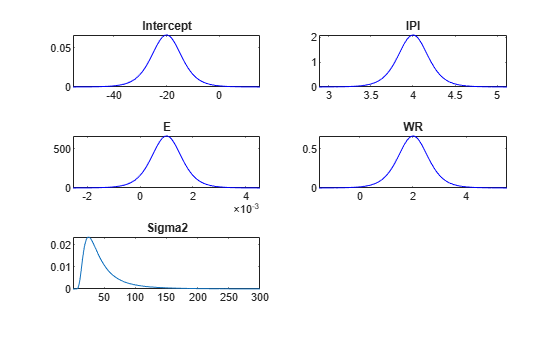`plot` replaces the current distribution figure with a plot of the prior distribution of the disturbance variance.

Load the Nelson-Plosser data set, and create variables for the predictor and response data.

```load Data_NelsonPlosser X = DataTable{:,PriorMdl.VarNames(2:end)}; y = DataTable.GNPR;```

Estimate the posterior distributions.

`PosteriorMdl = estimate(PriorMdl,X,y,'Display',false);`

`PosteriorMdl` is a `conjugateblm` model object that contains the posterior distributions of $\beta$ and ${\sigma }^{2}$.

Plot the posterior distributions.

`plot(PosteriorMdl);`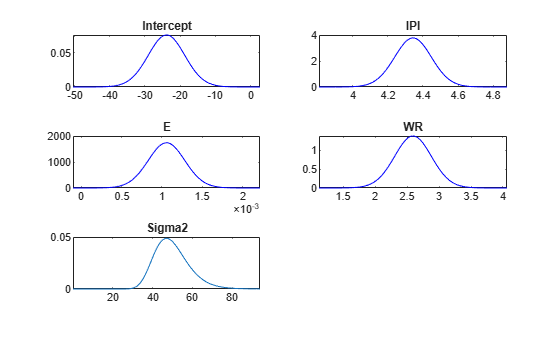Plot the prior and posterior distributions of the parameters on the same subplots.

`plot(PosteriorMdl,PriorMdl);`Consider the regression model in Plot Prior and Posterior Distributions.

Load the Nelson-Plosser data set, create a default conjugate prior model, and then estimate the posterior using the first 75% of the data. Turn off the estimation display.

```p = 3; VarNames = ["IPI" "E" "WR"]; PriorMdl = bayeslm(p,'ModelType','conjugate','VarNames',VarNames); load Data_NelsonPlosser X = DataTable{:,PriorMdl.VarNames(2:end)}; y = DataTable.GNPR; d = 0.75; PosteriorMdlFirst = estimate(PriorMdl,X(1:floor(d*end),:),y(1:floor(d*end)),... 'Display',false);```

Plot the prior distribution and the posterior distribution of the disturbance variance. Return the figure handle.

`[~,~,~,h] = plot(PosteriorMdlFirst,PriorMdl,'VarNames','Sigma2');`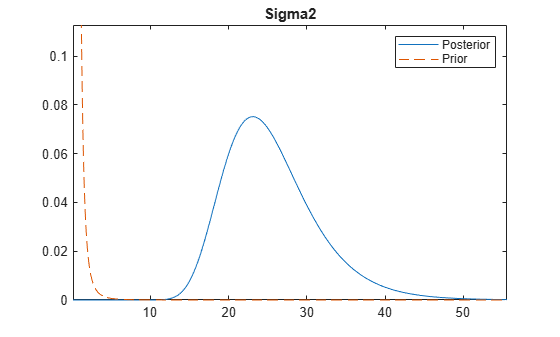`h` is the figure handle for the distribution plot. If you change the tag name of the figure by changing the `Tag` property, then the next `plot` call places all new distribution plots on a different figure.

Change the name of the figure handle to `FirstHalfData` using dot notation.

`h.Tag = 'FirstHalfData';`

Estimate the posterior distribution using the rest of the data. Specify the posterior distribution based on the final 25% of the data as the prior distribution.

```PosteriorMdl = estimate(PosteriorMdlFirst,X(ceil(d*end):end,:),... y(ceil(d*end):end),'Display',false);```

Plot the posterior of the disturbance variance based on half of the data and all the data to a new figure.

`plot(PosteriorMdl,PosteriorMdlFirst,'VarNames','Sigma2');`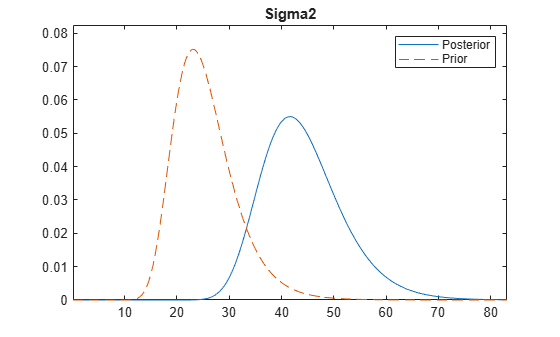This type of plot shows the evolution of the posterior distribution when you incorporate new data.

Consider the regression model in Plot Prior and Posterior Distributions.

Load the Nelson-Plosser data set and create a default conjugate prior model.

```p = 3; VarNames = ["IPI" "E" "WR"]; PriorMdl = bayeslm(p,'ModelType','conjugate','VarNames',VarNames); load Data_NelsonPlosser X = DataTable{:,PriorMdl.VarNames(2:end)}; y = DataTable.GNPR;```

Plot the prior distributions. Request the values of the parameters used to create the plots and their respective densities.

`[pointsUsedPrior,priorDensities1] = plot(PriorMdl);``pointsUsedPrior` is a 5-by-1 cell array of 1-by-1000 numeric vectors representing the values of the parameters that `plot` uses to plot the corresponding densities. The first element corresponds to the intercept, the next three elements correspond to the regression coefficients, and the last element corresponds to the disturbance variance. `priorDensities1` has the same dimensions as `pointsUsed` and contains the corresponding density values.

Estimate the posterior distribution. Turn off the estimation display.

`PosteriorMdl = estimate(PriorMdl,X,y,'Display',false);`

Plot the posterior distributions. Request the values of the parameters used to create the plots and their respective densities.

`[pointsUsedPost,posteriorDensities1] = plot(PosteriorMdl);`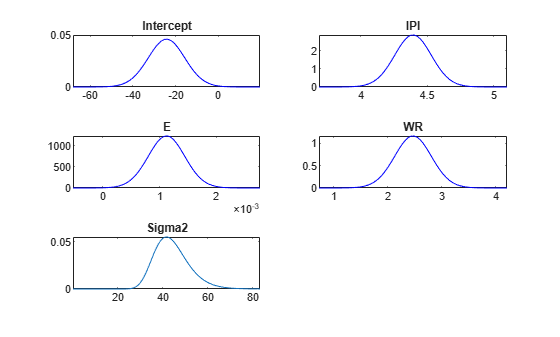`pointsUsedPost` and `posteriorDensities1` have the same dimensions as `pointsUsedPrior`. The `pointsUsedPost` output can be different from `pointsUsedPrior`. `posteriorDensities1` contains the posterior density values.

Plot the prior and posterior distributions. Request the values of the parameters used to create the plots and their respective densities.

`[pointsUsedPP,posteriorDensities2,priorDensities2] = plot(PosteriorMdl,PriorMdl);`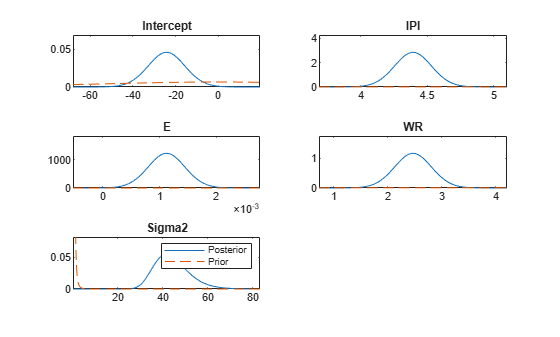All output values have the same dimensions as `pointsUsedPrior`. The `posteriorDensities2` output contains the posterior density values. The `priorDensities2` output contains the prior density values.

Confirm that `pointsUsedPP` is equal to `pointsUsedPost`.

```compare = @(a,b)sum(a == b) == numel(a); cellfun(compare,pointsUsedPost,pointsUsedPP)```
```ans = 5x1 logical array 1 1 1 1 1 ```

The points used are equivalent.

Confirm that the posterior densities are the same, but that the prior densities are not.

`cellfun(compare,posteriorDensities1,posteriorDensities2)`
```ans = 5x1 logical array 1 1 1 1 1 ```
`cellfun(compare,priorDensities1,priorDensities2)`
```ans = 5x1 logical array 0 0 0 0 0 ```

When plotting only the prior distribution, `plot` evaluates the prior densities at points that produce a clear plot of the prior distribution. When plotting both a prior and posterior distribution, `plot` prefers to plot the posterior clearly. Therefore, plot can determine a different set of points to use.

Consider the regression model in Plot Prior and Posterior Distributions.

Load the Nelson-Plosser data set and create a default conjugate prior model for the regression coefficients and disturbance variance. Then, estimate the posterior distribution and obtain the estimation summary table from `summarize`.

```p = 3; VarNames = ["IPI" "E" "WR"]; PriorMdl = bayeslm(p,'ModelType','conjugate','VarNames',VarNames); load Data_NelsonPlosser X = DataTable{:,PriorMdl.VarNames(2:end)}; y = DataTable.GNPR; PosteriorMdl = estimate(PriorMdl,X,y);```
```Method: Analytic posterior distributions Number of observations: 62 Number of predictors: 4 Log marginal likelihood: -259.348 | Mean Std CI95 Positive Distribution ----------------------------------------------------------------------------------- Intercept | -24.2494 8.7821 [-41.514, -6.985] 0.003 t (-24.25, 8.65^2, 68) IPI | 4.3913 0.1414 [ 4.113, 4.669] 1.000 t (4.39, 0.14^2, 68) E | 0.0011 0.0003 [ 0.000, 0.002] 1.000 t (0.00, 0.00^2, 68) WR | 2.4683 0.3490 [ 1.782, 3.154] 1.000 t (2.47, 0.34^2, 68) Sigma2 | 44.1347 7.8020 [31.427, 61.855] 1.000 IG(34.00, 0.00069) ```
```summaryTbl = summarize(PosteriorMdl); summaryTbl = summaryTbl.MarginalDistributions;```

`summaryTbl` is a table containing the statistics that `estimate` displays at the command line.

For each parameter, determine a set of 50 evenly spaced values within three standard deviations of the mean. Put the values into the cells of a 5-by-1 cell vector following the order of the parameters that comprise the rows of the estimation summary table.

```Points = cell(numel(summaryTbl.Mean),1); % Preallocation for j = 1:numel(summaryTbl.Mean) Points{j} = linspace(summaryTbl.Mean(j) - 3*summaryTbl.Std(j),... summaryTbl.Mean(j) + 2*summaryTbl.Std(j),50); end```

Plot the posterior distributions within their respective intervals.

`plot(PosteriorMdl,'Points',Points)`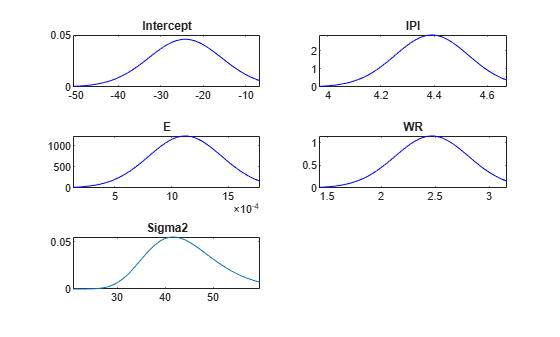## Input Arguments

collapse all

Bayesian linear regression model storing posterior distribution characteristics, specified as a `conjugateblm` or `empiricalblm` model object returned by `estimate`.

When you also specify `PriorMdl`, then `PosteriorMdl` is the posterior distribution composed of `PriorMdl` and data. If the `NumPredictors` and `VarNames` properties of the two models are not equal, `plot` issues an error.

Bayesian linear regression model storing prior distribution characteristics, specified as a `conjugateblm`, `semiconjugateblm`, `diffuseblm`, `empiricalblm`, `customblm`, `mixconjugateblm`, `mixsemiconjugateblm`, or `lassoblm` model object returned by `bayeslm`.

When you also specify `PosteriorMdl`, then `PriorMdl` is the prior distribution that, when combined with the data likelihood, forms `PosteriorMdl`. If the `NumPredictors` and `VarNames` properties of the two models are not equal, `plot` issues an error.

### Name-Value Arguments

Specify optional pairs of arguments as `Name1=Value1,...,NameN=ValueN`, where `Name` is the argument name and `Value` is the corresponding value. Name-value arguments must appear after other arguments, but the order of the pairs does not matter.

Before R2021a, use commas to separate each name and value, and enclose `Name` in quotes.

Example: `'VarNames',["Beta1"; "Beta2"; "Sigma2"]` plots the distributions of regression coefficients corresponding to the names `Beta1` and `Beta2` in the `VarNames` property of the model object and the disturbance variance `Sigma2`.

Parameter names indicating which densities to plot in the figure, specified as the comma-separated pair consisting of `'VarNames'` and a cell vector of character vectors or string vector. `VarNames` must include `"Intercept"`, any name in the `VarNames` property of `PriorMdl` or `PosteriorMdl`, or `"Sigma2"`.

By default, `plot` chooses `"Intercept"` (if an intercept exists in the model), all regression coefficients, and `"Sigma2"`. If the model has more than 34 regression coefficients, then `plot` chooses the first through the 34th only.

`VarNames` is case insensitive.

Tip

If your model contains many variables, then try plotting subsets of the parameters on separate plots for a better view of the distributions.

Example: `'VarNames',["Beta(1)","Beta(2)"]`

Data Types: `string` | `cell`

Parameter values for density evaluation and plotting, specified as the comma-separated pair consisting of `'Points'` and a `numPoints`-dimensional numeric vector or a `numVarNames`-dimensional cell vector of numeric vectors. `numPoints` is the number of parameters values that `plot` evaluates and plots the density.

• If `Points` is a numeric vector, then `plot` evaluates and plots the densities of all specified distributions by using its elements (see `VarNames`).

• If `Points` is a cell vector of numeric vectors, then:

• `numVarNames` must be `numel(VarNames)`, where `VarNames` is the value of `VarNames`.

• Cells correspond to the elements of `VarNames`.

• For `j` = 1,…,`numVarNames`, `plot` evaluates and plots the density of the parameter named `VarNames{j}` by using the vector of points in cell `Points(j)`.

By default, `plot` determines `1000` adequate values at which to evaluate and plot the density for each parameter.

Example: ```'Points',{1:0.1:10 10:0.2:25 1:0.01:2}```

Data Types: `double` | `cell`

## Output Arguments

collapse all

Parameter values used for density evaluation and plotting, returned as a cell vector of numeric vectors.

Suppose `Points` and `VarNames` are the values of `Points` and `VarNames`, respectively. If `Points` is a numeric vector, then `PointsUsed` is `repmat({Points},numel(VarNames))`. Otherwise, `PointsUsed` equals `Points`. Cells correspond to the names in `VarNames`.

Evaluated and plotted posterior densities, returned as a `numVarNames`-by-1 cell vector of numeric row vectors. `numVarNames` is `numel(VarNames)`, where `VarNames` is the value of `VarNames`. Cells correspond to the names in `VarNames`. `posteriorDensity` has the same dimensions as `priorDensity`.

Evaluated and plotted prior densities, returned as a `numVarNames`-by-1 cell vector of numeric row vectors. `priorDensity` has the same dimensions as `posteriorDensity`.

Figure window containing distributions, returned as a `figure` object.

`plot` overwrites the figure window that it produces.

If you rename `FigureHandle` for a new figure window, or call `figure` before calling `plot`, then `plot` continues to overwrite the current figure. To plot distributions to a different figure window, change the figure identifier of the current figure window by renaming its `Tag` property. For example, to rename the current figure window called `FigureHandle` to `newFigure`, at the command line, enter:

`FigureHandle.Tag = newFigure;`

## Limitations

Because improper distributions (distributions with densities that do not integrate to 1) are not well defined, `plot` cannot plot them very well.

collapse all

### Bayesian Linear Regression Model

A Bayesian linear regression model treats the parameters β and σ2 in the multiple linear regression (MLR) model yt = xtβ + εt as random variables.

For times t = 1,...,T:

• yt is the observed response.

• xt is a 1-by-(p + 1) row vector of observed values of p predictors. To accommodate a model intercept, x1t = 1 for all t.

• β is a (p + 1)-by-1 column vector of regression coefficients corresponding to the variables that compose the columns of xt.

• εt is the random disturbance with a mean of zero and Cov(ε) = σ2IT×T, while ε is a T-by-1 vector containing all disturbances. These assumptions imply that the data likelihood is

`$\ell \left(\beta ,{\sigma }^{2}|y,x\right)=\prod _{t=1}^{T}\varphi \left({y}_{t};{x}_{t}\beta ,{\sigma }^{2}\right).$`

ϕ(yt;xtβ,σ2) is the Gaussian probability density with mean xtβ and variance σ2 evaluated at yt;.

Before considering the data, you impose a joint prior distribution assumption on (β,σ2). In a Bayesian analysis, you update the distribution of the parameters by using information about the parameters obtained from the likelihood of the data. The result is the joint posterior distribution of (β,σ2) or the conditional posterior distributions of the parameters.

## Version History

Introduced in R2017a